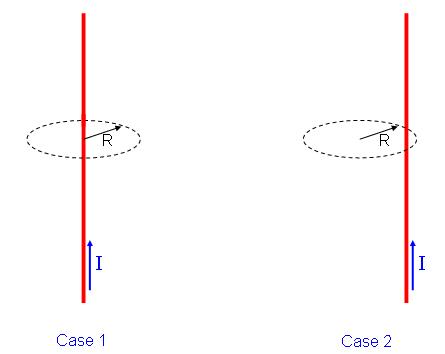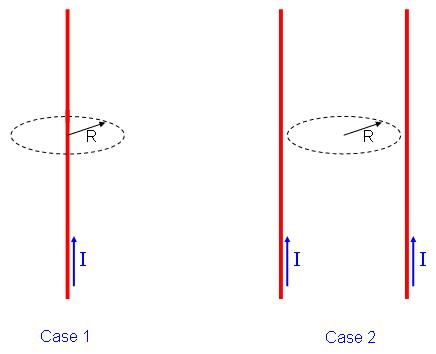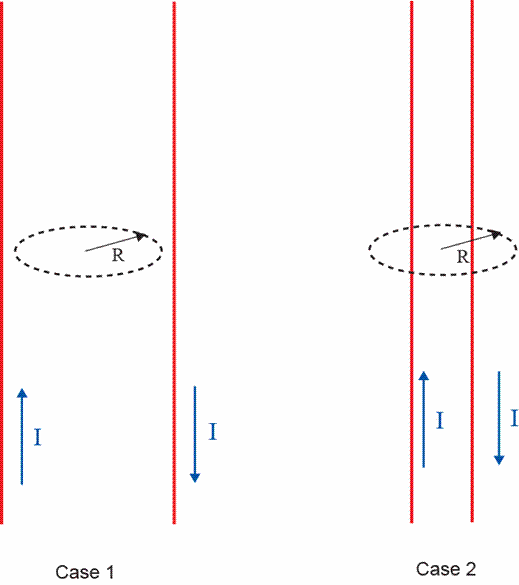# Problem: Part ATwo loops are placed near-identical current carrying wires as shown in Case 1 and Case 2 below.For which loop is ∫B·dl greater?A. Case 1B. Case 2C. The integral is the same for both CasesPart BTwo loops are placed near current carrying wires as shown in Case 1 and Case 2 below.For which loop is ∫B·dl greater?A. Case 1B. Case 2C. The integral is the same for both CasesBriefly explain your reasoningPart BTwo loops are placed near current carrying wires as shown in Case 1 and Case 2 below. In both cases the direction of the current in the two wires are opposite to each other.For which loop is ∫B·dl greater?A. Case 1B. Case 2C. The integral is the same for both Cases

###### FREE Expert Solution

Ampere's law:

$\overline{){\mathbf{\oint }}{\mathbf{B}}{\mathbf{·}}{\mathbf{dl}}{\mathbf{=}}{{\mathbf{\mu }}}_{{\mathbf{0}}}{{\mathbf{I}}}_{\mathbf{e}\mathbf{n}\mathbf{c}\mathbf{l}\mathbf{o}\mathbf{s}\mathbf{e}\mathbf{d}}}$

96% (115 ratings)###### Problem Details

Part ATwo loops are placed near-identical current carrying wires as shown in Case 1 and Case 2 below.

For which loop is ∫B·dl greater?

A. Case 1

B. Case 2

C. The integral is the same for both Cases

Part B

Two loops are placed near current carrying wires as shown in Case 1 and Case 2 below.For which loop is ∫B·dl greater?

A. Case 1

B. Case 2

C. The integral is the same for both Cases

Part B

Two loops are placed near current carrying wires as shown in Case 1 and Case 2 below. In both cases the direction of the current in the two wires are opposite to each other.For which loop is ∫B·dl greater?

A. Case 1

B. Case 2

C. The integral is the same for both Cases